# Geometry

Geometry is all about shapes and their properties.

If you like playing with objects, or like drawing, then geometry is for you!

Geometry can be divided into:Plane Geometry is about flat shapes like lines, circles and triangles ... shapes that can be drawn on a piece of paper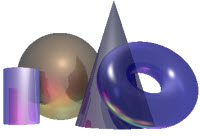Solid Geometry is about three dimensional objects like cubes, prisms, cylinders and spheres.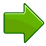Hint: Try drawing some of the shapes and angles as you learn ... it helps.

## Point, Line, Plane and SolidA Point has no dimensions, only position
A Line is one-dimensional
A Plane is two dimensional (2D)
A Solid is three-dimensional (3D)

## Why?

Why do we do Geometry? To discover patterns, find areas, volumes, lengths and angles, and better understand the world around us.

## Plane Geometry

Plane Geometry is all about shapes on a flat surface (like on an endless piece of paper).### Polygons

A Polygon is a 2-dimensional shape made of straight lines. Triangles and Rectangles are polygons.

Here are some more:PentagonPentagramHexagon### Symbols

There are many special symbols used in Geometry. Here is a short reference for you:

Geometric Symbols

### Angles

Types of AnglesAcute Angles Right Angles Obtuse Angles Straight Angle Reflex Angles Full Rotation

### Using Drafting Tools### Transformations and Symmetry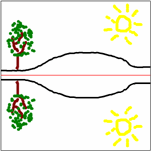### Coordinates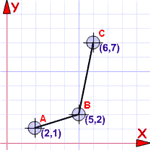## More Advanced Topics in Plane Geometry

### Pythagoras### Conic Sections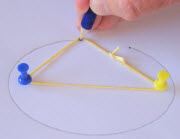Triangle Centers

## TrigonometryTrigonometry is a special subject of its own, so you might like to visit:

## Solid Geometry

Solid Geometry is the geometry of three-dimensional space - the kind of space we live in ...Common 3D Shapes

### Polyhedra and Non-Polyhedra

There are two main types of solids, "Polyhedra", and "Non-Polyhedra":

Polyhedra (they must have flat faces):

Non-Polyhedra (when any surface is not flat):Sphere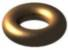TorusCylinder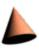Cone